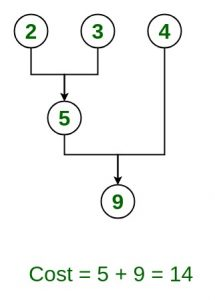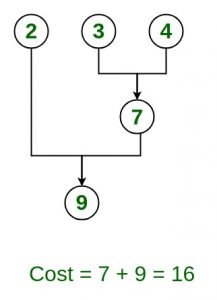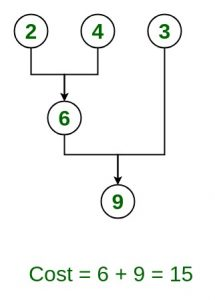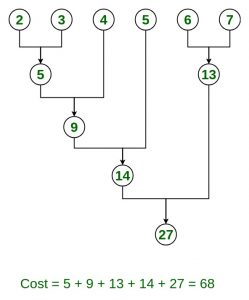Open in App
Not now

# Optimal File Merge Patterns

• Difficulty Level : Medium
• Last Updated : 15 Jun, 2022

Given n number of sorted files, the task is to find the minimum computations done to reach the Optimal Merge Pattern.
When two or more sorted files are to be merged altogether to form a single file, the minimum computations are done to reach this file are known as Optimal Merge Pattern.

If more than 2 files need to be merged then it can be done in pairs. For example, if need to merge 4 files A, B, C, D. First Merge A with B to get X1, merge X1 with C to get X2, merge X2 with D to get X3 as the output file.

If we have two files of sizes m and n, the total computation time will be m+n. Here, we use the greedy strategy by merging the two smallest size files among all the files present.

Examples:
Given 3 files with sizes 2, 3, 4 units. Find an optimal way to combine these files

Input: n = 3, size = {2, 3, 4}
Output: 14
Explanation: There are different ways to combine these files:
Method 1: Optimal methodMethod 2:Method 3:Input: n = 6, size = {2, 3, 4, 5, 6, 7}
Output: 68
Explanation: Optimal way to combine these filesInput: n = 5, size = {5,10,20,30,30}
Output: 205

Input: n = 5, size = {8,8,8,8,8}
Output: 96

Observations:

From the above results, we may conclude that for finding the minimum cost of computation we need to have our array always sorted, i.e., add the minimum possible computation cost and remove the files from the array. We can achieve this optimally using a min-heap(priority-queue) data structure.
Approach:

Node represents a file with a given size also given nodes are greater than 2

1. Add all the nodes in a priority queue (Min Heap).{pq.poll = file size}
2. Initialize count = 0 // variable to store file computations.
3. Repeat while (size of priority Queue is greater than 1)
1. int weight = pq.poll(); pq.pop;//pq denotes priority queue, remove 1st smallest and pop(remove) it out
2. weight+=pq.poll()  && pq.pop(); // add the second element and then pop(remove) it out
3. count +=weight;
4. count is the final answer

Below is the implementation of the above approach:

## C++

 `// C++ program to implement``// Optimal File Merge Pattern``#include ``using` `namespace` `std;` `// Function to find minimum computation``int` `minComputation(``int` `size, ``int` `files[])``{` `    ``// Create a min heap``    ``priority_queue<``int``, vector<``int``>, greater<``int``> > pq;` `    ``for` `(``int` `i = 0; i < size; i++) {` `        ``// Add sizes to priorityQueue``        ``pq.push(files[i]);``    ``}` `    ``// Variable to count total Computation``    ``int` `count = 0;` `    ``while` `(pq.size() > 1) {` `        ``// pop two smallest size element``        ``// from the min heap``        ``int` `first_smallest = pq.top();``        ``pq.pop();``        ``int` `second_smallest = pq.top();``        ``pq.pop();` `        ``int` `temp = first_smallest + second_smallest;` `        ``// Add the current computations``        ``// with the previous one's``        ``count += temp;` `        ``// Add new combined file size``        ``// to priority queue or min heap``        ``pq.push(temp);``    ``}``    ``return` `count;``}` `// Driver code``int` `main()``{` `    ``// No of files``    ``int` `n = 6;` `    ``// 6 files with their sizes``    ``int` `files[] = { 2, 3, 4, 5, 6, 7 };` `    ``// Total no of computations``    ``// do be done final answer``    ``cout << ``"Minimum Computations = "``         ``<< minComputation(n, files);` `    ``return` `0;``}` `// This code is contributed by jaigoyal1328`

## Java

 `// Java program to implement``// Optimal File Merge Pattern` `import` `java.util.PriorityQueue;``import` `java.util.Scanner;` `public` `class` `OptimalMergePatterns {` `    ``// Function to find minimum computation``    ``static` `int` `minComputation(``int` `size, ``int` `files[])``    ``{` `        ``// create a min heap``        ``PriorityQueue pq = ``new` `PriorityQueue<>();` `        ``for` `(``int` `i = ``0``; i < size; i++) {` `            ``// add sizes to priorityQueue``            ``pq.add(files[i]);``        ``}` `        ``// variable to count total computations``        ``int` `count = ``0``;` `        ``while` `(pq.size() > ``1``) {` `            ``// pop two smallest size element``            ``// from the min heap``            ``int` `temp = pq.poll() + pq.poll();` `            ``// add the current computations``            ``// with the previous one's``            ``count += temp;` `            ``// add new combined file size``            ``// to priority queue or min heap``            ``pq.add(temp);``        ``}` `        ``return` `count;``    ``}` `    ``public` `static` `void` `main(String[] args)``    ``{` `        ``// no of files``        ``int` `size = ``6``;` `        ``// 6 files with their sizes``        ``int` `files[] = ``new` `int``[] { ``2``, ``3``, ``4``, ``5``, ``6``, ``7` `};` `        ``// total no of computations``        ``// do be done final answer``        ``System.out.println(``"Minimum Computations = "``                           ``+ minComputation(size, files));``    ``}``}`

## Python3

 `# Python Program to implement``# Optimal File Merge Pattern`  `class` `Heap():``    ``# Building own implementation of Min Heap``    ``def` `__init__(``self``):` `        ``self``.h ``=` `[]` `    ``def` `parent(``self``, index):``        ``# Returns parent index for given index` `        ``if` `index > ``0``:``            ``return` `(index ``-` `1``) ``/``/` `2` `    ``def` `lchild(``self``, index):``        ``# Returns left child index for given index` `        ``return` `(``2` `*` `index) ``+` `1` `    ``def` `rchild(``self``, index):``        ``# Returns right child index for given index` `        ``return` `(``2` `*` `index) ``+` `2` `    ``def` `addItem(``self``, item):` `        ``# Function to add an item to heap``        ``self``.h.append(item)` `        ``if` `len``(``self``.h) ``=``=` `1``:` `            ``# If heap has only one item no need to heapify``            ``return` `        ``index ``=` `len``(``self``.h) ``-` `1``        ``parent ``=` `self``.parent(index)` `        ``# Moves the item up if it is smaller than the parent``        ``while` `index > ``0` `and` `item < ``self``.h[parent]:``            ``self``.h[index], ``self``.h[parent] ``=` `self``.h[parent], ``self``.h[parent]``            ``index ``=` `parent``            ``parent ``=` `self``.parent(index)` `    ``def` `deleteItem(``self``):` `        ``# Function to add an item to heap``        ``length ``=` `len``(``self``.h)``        ``self``.h[``0``], ``self``.h[length``-``1``] ``=` `self``.h[length``-``1``], ``self``.h[``0``]``        ``deleted ``=` `self``.h.pop()` `        ``# Since root will be violating heap property``        ``# Call moveDownHeapify() to restore heap property``        ``self``.moveDownHeapify(``0``)` `        ``return` `deleted` `    ``def` `moveDownHeapify(``self``, index):` `        ``# Function to make the items follow Heap property``        ``# Compares the value with the children and moves item down` `        ``lc, rc ``=` `self``.lchild(index), ``self``.rchild(index)``        ``length, smallest ``=` `len``(``self``.h), index` `        ``if` `lc < length ``and` `self``.h[lc] <``=` `self``.h[smallest]:``            ``smallest ``=` `lc` `        ``if` `rc < length ``and` `self``.h[rc] <``=` `self``.h[smallest]:``            ``smallest ``=` `rc` `        ``if` `smallest !``=` `index:``            ``# Swaps the parent node with the smaller child``            ``self``.h[smallest], ``self``.h[index] ``=` `self``.h[index], ``self``.h[smallest]` `            ``# Recursive call to compare next subtree``            ``self``.moveDownHeapify(smallest)` `    ``def` `increaseItem(``self``, index, value):``        ``# Increase the value of 'index' to 'value'` `        ``if` `value <``=` `self``.h[index]:``            ``return` `        ``self``.h[index] ``=` `value``        ``self``.moveDownHeapify(index)`  `class` `OptimalMergePattern():``    ``def` `__init__(``self``, n, items):` `        ``self``.n ``=` `n``        ``self``.items ``=` `items``        ``self``.heap ``=` `Heap()` `    ``def` `optimalMerge(``self``):` `        ``# Corner cases if list has no more than 1 item``        ``if` `self``.n <``=` `0``:``            ``return` `0` `        ``if` `self``.n ``=``=` `1``:``            ``return` `self``.items[``0``]` `        ``# Insert items into min heap``        ``for` `_ ``in` `self``.items:``            ``self``.heap.addItem(_)` `        ``count ``=` `0``        ``while` `len``(``self``.heap.h) !``=` `1``:``            ``tmp ``=` `self``.heap.deleteItem()``            ``count ``+``=` `(tmp ``+` `self``.heap.h[``0``])``            ``self``.heap.increaseItem(``0``, tmp ``+` `self``.heap.h[``0``])` `        ``return` `count`  `# Driver Code``if` `__name__ ``=``=` `'__main__'``:``    ``OMP ``=` `OptimalMergePattern(``6``, [``2``, ``3``, ``4``, ``5``, ``6``, ``7``])``    ``ans ``=` `OMP.optimalMerge()``    ``print``(ans)` `# This code is contributed by Rajat Gupta`

Output

`Minimum Computations = 68`

Time Complexity: O(nlogn)
Auxiliary Space: O(n)

My Personal Notes arrow_drop_up